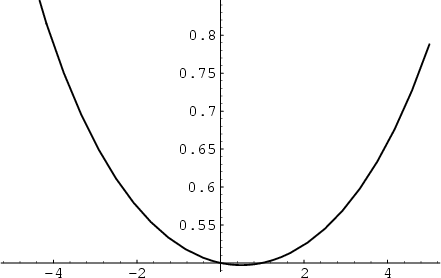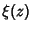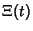## Xi Function(1)

whereis the Riemann Zeta Function andis the Gamma Function (Gradshteyn and Ryzhik 1980, p. 1076). Thefunction satisfies the identity(2)

The zeros ofand of its Derivatives are all located on the Critical Strip, where. Therefore, the nontrivial zeros of the Riemann Zeta Function exactly correspond to those of. The functionis related to what Gradshteyn and Ryzhik (1980, p. 1074) callby(3)

where. This function can also be defined as(4)

giving(5)

The de Bruijn-Newman Constant is defined in terms of thefunction.